# Samacheer Kalvi 8th Maths Solutions Term 2 Chapter 3 Geometry Ex 3.1

Students can Download Maths Chapter 3 Geometry Ex 3.1 Questions and Answers, Notes Pdf, Samacheer Kalvi 8th Maths Book Solutions Guide Pdf helps you to revise the complete Tamilnadu State Board New Syllabus and score more marks in your examinations.

## Tamilnadu Samacheer Kalvi 8th Maths Solutions Term 2 Chapter 3 Geometry Ex 3.1

Question 1.
Fill in the blanks:

Question (i)
If in a ∆ PQR, PR2 = PQ2 + QR2, then the right angle of ∆ PQR is at the vertex ………
Q
Hint:Question:
(ii) If ‘l’ and ‘m’ are the legs and is the hypotenuse of a right angled triangle then, l2 = ……….
n2 – m2
Hint:Question (iii)
If the sides of a triangle are in the ratio 5:12:13 then, it is ………
a right angled triangle.
132 = 169
52 = 25
122 = 144
169 = 25 + 144
132 = 52 + 122
By Pythagoras theorem, In a right triangle, square of the hypotenuse is equal to the sum of the squares of other two sides.Question (iv)
If a perpendicular is drawn to the hypotenuse of a right angled triangle, then each of the three pairs of triangles formed are …………
Similar.

Question (v)
In the figure BE2 = TE x ………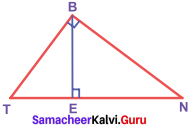EN

Question 2.
Say True or False.

Question (i)
8, 15, 17 is a Pythagorean triplet.
True
Hint:
172 = 289
152 = 225
82 = 64
64 + 225 = 289 ⇒ 172 = 152 + 82

Question (ii)
In a right angled triangle, the hypotenuse is the greatest side.
False
Hint: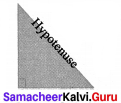Question (iii)
One of the legs of a right angled triangle PQR having ∠R = 90° is PQ.
False
Hint: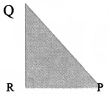In ∆ PQR, QR and RP are legs and PQ is the hypotenuse

Question (iv)
The hypotenuse of a right angled triangle whose sides are 9 and 40 is 49.
False
Hint: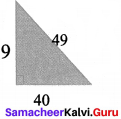492 = 2401
92 = 81
402 = 1600
402 + 92 = 1600 + 81 + 1681
492 = 2401
2401 ≠ 1681Question (v)
Pythagoras theorem is true for all types of triangles.
False
Hint:
Pyhtagoras theorem is true for only right angled triangles.

Question 3.
Check whether given sides are the sides of right – angled triangles, using Pythagoras theorem,

1. 8, 15, 17
2. 12, 13, 15
3. 30, 40, 50
4. 9, 40, 41
5. 24, 45, 51

Solution:
1. 8, 15, 17
Take a = 8,
b = 15 and
c = 17
Now a2 + b2 = 82 + 152 = 64 + 225 = 289
172 = 289 = c2
∴ a2 + b2 = c2
By the converse of Pythagoras theorem, the triangle with given measures is a right angled triangle.
yes.

2. 12, 13, 15
Take a = 12
b = 13 and
c = 15
Now a2 + b2 = 122 + 132 = 144 + 169 = 313
152 = 225 ≠ 313
By the converse of Pythagoras theorem, the triangle with given measures is not a right angled triangle.
No.

3. 30, 40, 50
Take a = 30
b = 40 and
c = 50
Now a2 + b2 = 302 + 402 = 900 + 1600 = 2500
c2 = 502 = 2500
∴ a2 + b2 = c2
By the converse of Pythagoras theorem, the triangle with given measures is a right angled triangle.
yes.

4. 9, 40, 41
Take a = 9
b = 40 and
c = 41
Now a2 + b2 = 92 + 402 = 81 + 1600= 1681
c2 = 412 = 1681
∴ a2 + b2 = c2
By the converse of Pythagoras theorem, the triangle with given measures is a right angled triangle.
Yes.

5. 24, 45, 51
Take a = 24
b = 45 and
c = 51 Now
a2 + b2 = 242 + 452 = 576 + 2025 = 2601
c2 = 512 = 2601
a2 + b2 = c2
By the converse of Pyhtagoreas theorem, the triangle with given measure is a right angled triangle.
Yes.Question 4.
Find the unknown side in the following triangles.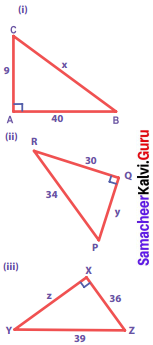Solution:
(i) From ∆ ABC, by Pythagoras theorem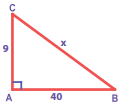BC2 = AB2 + AC2
Take AB2 + AC2 = 92 + 402 = 81 + 1600 = 1681
BC2 = AB2 + AC2 = 1681 = 412
BC2 = 412 ⇒ BC = 41
∴ x = 41

(ii) From ∆ PQR, by Pythagoras theorem,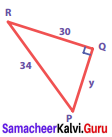PR2 = PQ2 + QR2
342 = y2 + 302
⇒ y2 = 342 – 302
= 1156 – 900
= 256 = 162
y2 = 162 ⇒ y = 16

(iii) From ∆ XYZ, by Pythagoras theorem,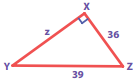YZ2 = XY2 + XZ2
⇒ XY2 = YZ2 – XZ2
Z2 = 392 – 362
= 1521 – 1296 = 225 = 152
Z2 = 152
⇒ Z = 15

Question 5.
An isosceles triangle has equal sides each 13 cm and a base 24 cm in length. Find its height.
Solution:
In an isosceles triangle the altitude dives its base into two equal parts. Now in the figure, ∆ ABC is an isosceles triangle with AD as its height.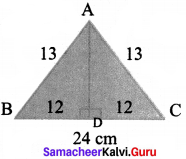In the figure, AD is the altitude and Δ ABD is a right triangle.
By Pythagoras theorem,
⇒ AD2 = AB2 – BD2
= 132 – 122 = 169 – 144 = 25

Question 6.
In the figure, find PR and QR.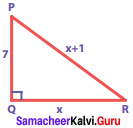Solution:
In the figure ∆ PQR is a right triangle.
By Pythagoras theorem,
PR2 = PQ2 + QR2
(x + 1)2 = 72 + x2
x2 +2 × x × 1 + 12 = 49+ x2
2x + 1 = 49
2x = 49 – 1 = 48
x = $$\frac{48}{2}$$ = 24
∴ PR = x + 1 = 24 + 1 = 25
QR = x = 24Question 7.
The length and breadth of the screen of an LED – TV are 24 inches and 18 inches. Find the length of its diagonal.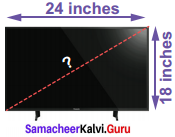Solution:
The length and breadth of a LED TV form a right angled triangle with its diagonal.
Therefore by Pythagoras theorem,
AC2 = AB2 + BC2
= 242 + 182 = 576 + 324 = 900 = 302
∴ AC = 30 ⇒ The length of the diagonal is 30 inches.

Question 8.
Find the distance between the helicopter and the ship.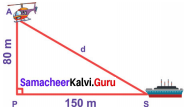Solution:
From the figure AS is the distance between the helicopter and the ship.
∆ APS is a right angled triangle, by Pythagoras theorem,
AS2 = AP2 + PS2
= 802 + 1502
= 6400 + 22500 = 28900 = 1702
∴ The distance between the helicopter and the ship is 170 m

Question 9.
From the figure, 1. If TA = 3 cm and OT = 6 cm, find TG.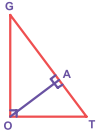Solution:
1. From the figure, if, TA = 3 cm, OT = 6 cm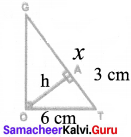By Pythagoras theorem,
OA2 = OT2 – TA2 = 62 – 32
i.e. h2 = 36 – 9 = 27 cm.
Now, by altitude – on – hypotenuse theorem
h2 = xy
27 = x × 3
x = $$\frac{27}{3}$$ = 9 cm
TG = x + 3 = 9 + 3 = 12 cm

Question 10.
If RQ = 15 cm and RP = 20 cm, find PQ, PS and SQ.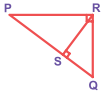Solution: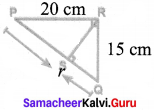RQ = 15cm
RP = 20 cm and ∆ PQR is a right angled triangle
By Pythagoras theorem, p 20 cm
PQ2 = PR2 + RQ2 = 202 + 152
= 400 + 225 = 625 = 252
∴ PQ = 25 cm
Now by altitude – on – hypotenuse theorem,
RQ2 = q x r
152 = q x 25
q = $$\frac{225}{25}$$ = 9 cm ⇒ SQ = 9 cm
PR2 = P x r
202 = P x 25
P = $$\frac{400}{25}$$ = 16 cm ⇒ PS = 16 cm

1. PQ = 25 cm
2. PS = 16 cm
3. SQ = 9 cm

Objective Type Questions

Question 11.
If ∆ GUT is isosceles and right angled, then ∠TUG is …………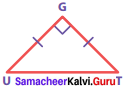(a) 30°
(b) 40°
(c) 45°
(d) 55°
(c) 45°
Hint:
∠U ∠T = 45° (∆ GUT is an isosceles given)
∴ ∠TUG = 45°Question 12.
The hypotenuse of a right angled triangle of sides 12 cm and 16 cm is ……….
(a) 28 cm
(b)20cm
(c) 24 cm
(d)21cm
(b) 20 cm
Hint:
Side take a = 12 cm
b = 16 cm
The hypotenuse c2 = a2 + b2 = 122 + 162
2 = 400 ⇒ c = 20 cm

Question 13.
The area of a rectangle of length 21 cm and diagonal 29 cm is ………. cm2
(a) 609
(b) 580
(c) 420
(d) 210
(c) 420
Hint: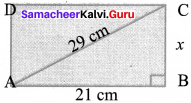Length = 21 cm
Diagonal = 29 cm
By the converse of Pythagoras theorem,
AB2 + BC2 = AC2
212 + x2 = 292
x2 = 841 – 441 = 400 = 202
x = 20 cm
Now area of the rectangle = length x breadth.
i.e. AB x BC = 21 cm x 20 cm = 420 cm2

Question 14.
if the square of the hypotenuse of an isosceles right triangle is 50 cm2, the length of each side is ………..
(c) 10 cm
(a) 25 cm
(b) 5 cm
(c) 10 cm
(d) 20 cm
(b) 5 cm
Hint:
By Pythagoras theorem
c2 = a2 + b2
In an isosceles triangle, a = b
c2 = a2 + a2 = 2a2
⇒ 2a2 = 50
a2 = 25 ⇒ a = 5cm
∴ The length of each sides a = 5cm, b = 5 cm.Question 15.
The sides of a right angled triangle are in the ratio 5 : 12 : 13 and its perimeter is 120 units then, the sides are .
(a) 25, 36, 59
(b) 10, 24, 26
(c) 36, 39, 45
(d) 20, 48, 52